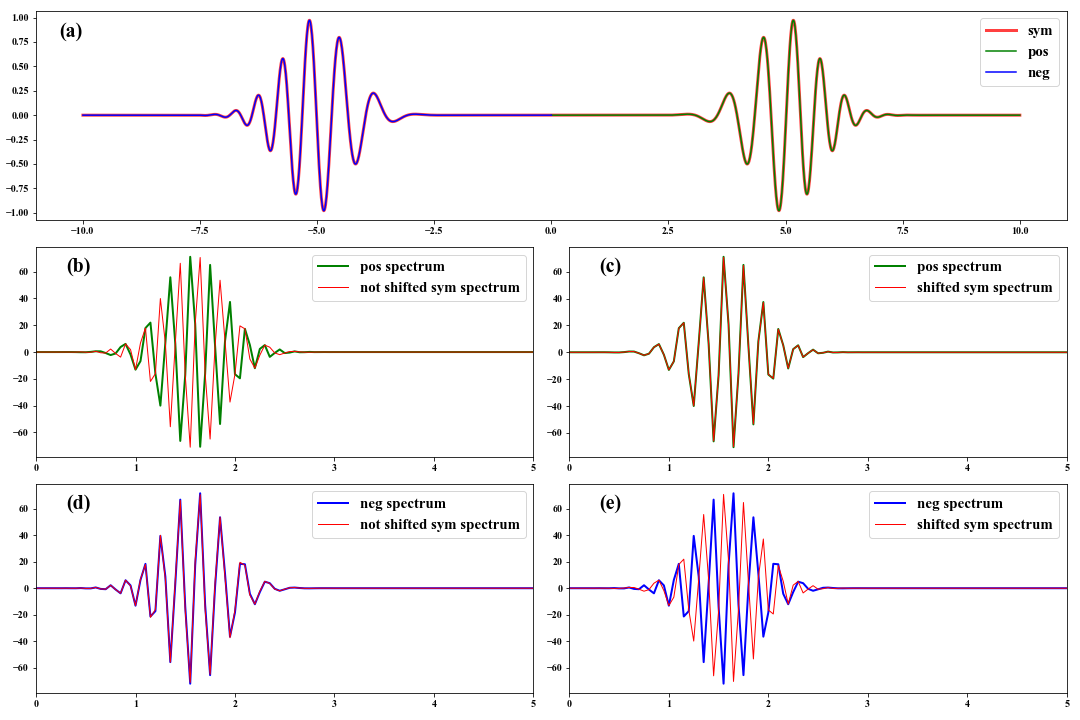## 1 Background

Ambient seismic noise cross-correlation has far-reaching implications for the passive seismic surface wave imaging from regional to continental scales (e.g. Campillo & Paul, Shapiro & Campillo, 2004). Spectral analysis of cross-correlations is the key to retrieving reliable dispersion information about surface waves. Based on the Fourier spectra of event seismic surface wave data, however, we need to shift the cross-correlation time function before doing FFT to obtain the right spectra.

## 2 A numerical example

Here, we generate a linear frequency modulated signal with decaying as $e^{-(t-t0)^2}$, and create a symmetric signal like cross-correlation functions extracted from seismic noise. The Fourier spectra separately from shifted and not shifted symmetric signal will be compared with the spectra of the positive and negative signals.

  1 2 3 4 5 6 7 8 9 10 11 12 13 14 15 16 17 18 19 20 21 22 23 24 25 26 27 28 29 30 31 32 33 34 35 36 37 38 39 40 41 42 43 44 45 46 47 48 49  n = 1001; nn = 2 * n - 1 t = np.linspace(0, 10, n) s = np.sin(t**2) * np.exp(-(t-5)**2) ss = np.zeros(nn) ss[:n] = s[::-1]; ss[n-1:] = s tt = np.linspace(-10, 10, len(ss)) f = np.arange(nn) / (t-t) / (nn-1) fd1 = np.fft.fft(s, nn) fd2 = np.fft.fft(s[::-1], nn) fdd1 = np.fft.fft(ss, nn) / 2 fdd2 = np.fft.fft(np.fft.fftshift(ss), nn) / 2 plt.figure(figsize=(15, 10)) plt.subplot(311) plt.plot(tt, ss, lw=3, color='r', alpha=0.75, label='sym') plt.plot(t, s, lw=1.5, color='g', label='pos') plt.plot(-t[::-1], s[::-1], lw=1.5, color='b', label='neg') plt.text(-10.5, 0.8, '(a)', fontdict={'size':20}) plt.legend(fontsize=15, loc='upper right') plt.subplot(323) plt.plot(f, fd1.real, lw=2, color='g', label='pos spectrum') plt.plot(f, fdd1.real, lw=1, color='r', label='not shifted sym spectrum') plt.legend(fontsize=15, loc='upper right') plt.text(0.3, 60, '(b)', fontdict={'size':20}) plt.xlim(0, 5) plt.subplot(324) plt.plot(f, fd1.real, lw=2, color='g', label='pos spectrum') plt.plot(f, fdd2.real, lw=1, color='r', label='shifted sym spectrum') plt.legend(fontsize=15, loc='upper right') plt.text(0.3, 60, '(c)', fontdict={'size':20}) plt.xlim(0, 5) plt.subplot(325) plt.plot(f, fd2.real, lw=2, color='b', label='neg spectrum') plt.plot(f, fdd1.real, lw=1, color='r', label='not shifted sym spectrum') plt.legend(fontsize=15, loc='upper right') plt.text(0.3, 60, '(d)', fontdict={'size':20}) plt.xlim(0, 5) plt.subplot(326) plt.plot(f, fd2.real, lw=2, color='b', label='neg spectrum') plt.plot(f, fdd2.real, lw=1, color='r', label='shifted sym spectrum') plt.legend(fontsize=15, loc='upper right') plt.text(0.3, 60, '(e)', fontdict={'size':20}) plt.xlim(0, 5) plt.show()## Bibliography

Campillo, M., & Paul, A. (2003). Long-range correlations in the diffuse seismic coda. Science, 299(5606), 547–549.

Shapiro, N. M., & Campillo, M. (2004). Emergence of broadband rayleigh waves from correlations of the ambient seismic noise. Geophysical Research Letters, 31(7).